Students can view and download the JEE Main 2021 Physics question paper with solutions for July 20 shift 1 here. The questions have been solved by our subject matter experts and the solutions are given in a detailed manner. This will enable students to quickly grasp the answer and understand the right approach to solve the problems. Students can directly view problems and solutions on this page. Additionally, this question paper is downloadable in PDF format.

In essence, JEE aspirants can make more productive use of their time by referring to the solved questions and solutions on this page. They can prepare more effectively and develop better problem-solving skills to crack some of the seemingly difficult problems. Candidates can gain higher confidence by solving the JEE Main Physics Question Paper on their own and boost their overall preparation for the entrance examination.

July 20 Shift 1 – Physics Question Paper and Solutions

Question 1. Consider the P-V diagram given below for a cyclic process. Find the net heat supplied to the system during the process.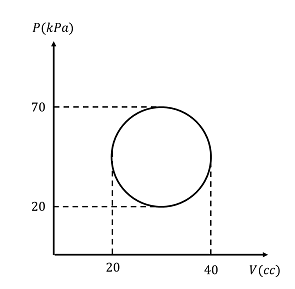a. 0.625п J
b. 0.25п J
c. 0.1п J
d. 0.2п J

It is a cyclic process so the net change in internal energy of the system will zero. i.e.,

ΔU = 0

From first law of thermodynamics,

ΔQ = ΔU + ΔW

Therefore, ΔQ = ΔW

ΔW is the area of the shaded region

Qcycle = Wcycle = π(25)(10) Kpa-cc

= π(25)(10) x 103 x 10-6

= 0.250 πJ

Question 2. A spring of force constant k = 100 N/m is compressed to x = 0.5 m by a block of mass 100 g released. Find the distance d where it falls.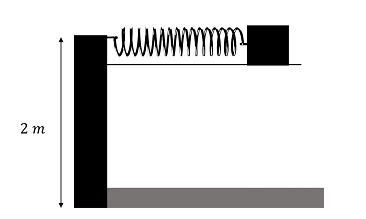a. 5 m
b. 10 m
c. 15 m
d. 20m

Here, we can find the horizontal velocity with which the block will leave the surface. So, using the principle of conservation of energy we have,

0 + (½)kx2 = (½)mv2 +0

Therefore,

$$\begin{array}{l}v=\sqrt{\frac{k}{m}}x\end{array}$$
—-(1)

Given k = 100 N/m

x = 0.5 m

m = 100 g

v = 5√10 m/s

Now, the horizontal distance moved by the block is given by d = vt, where t is the time taken by the block to fall the distance 2 m

Therefore, distance

$$\begin{array}{l}d=v\sqrt{\frac{2H}{g}}\end{array}$$
—-(2)

Substituting (1) in (2)

$$\begin{array}{l}d=5\sqrt{10}\sqrt{\frac{2H}{g}}\end{array}$$

Therefore,

$$\begin{array}{l}d=5\sqrt{10} \sqrt{\frac{2(2)}{10}}\end{array}$$

= 20 m

Question 3. In the given circuit find the current through 6Ω resistance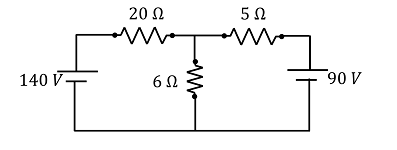a. 10 A
b. 7 A
c. 25 A
d. 30 A

Applying Kirchhoff’s law,

$$\begin{array}{l}\frac{V-140}{20}+\frac{V-0}{6}+\frac{V-90}{5}=0\end{array}$$

3(V-140)+10V+(V-90) =0

3V-420+10V+12V-1080 = 0

25V= 1500

V = 60

Current through 6Ω resistance, I = V/R = 60/6 = 10 A

Question 4. Four molecules of a diatomic gas are heated from 00C to 500C. Find the heat supplied to the gas if work done by it is zero.

a. 780 R
b. 500 R
c. 100 R
d. 650 R

For a diatomic molecule

Cv = 5R/2

Cp = 7R/2

Number of moles, n = 4

ΔT = 50

Sone Work done = 0, the process is isochoric

In an isochoric process, Q = ΔU = nCvΔT

Therefore, Q = (4)(5R/2)(50)

= 500 R

Question 5. For the spherical interface shown in the figure, the two different media with refractive indices n1 = 1.4 and n2 = 1.25 are present as shown. The image will be formed at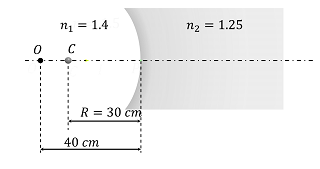a. -125/3
b. -50/6 cm
c. -25/2 cm
d. -20 cm

Given:

Position of the object (u)= -40 cm

Refractive index of medium 1(n1) = 1.4

Refractive index of medium 2 (n2) = 1.25

Radius of the interface (R) = -30 cm

We know that for the spherical interface,

$$\begin{array}{l}\frac{n_{2}}{v}-\frac{n_{1}}{u}=\frac{n_{2}-n_{1}}{R}\end{array}$$
$$\begin{array}{l}\frac{1.4}{v}-\frac{1.25}{-40}=\frac{1.4-1.25}{-30}\end{array}$$

V = -125/3

Question 6. When a disc slides on a smooth inclined surface from rest, the time taken to move from A to B is t1. When the disc performs pure rolling from rest then the time taken to move from A to B is t2. If

$$\begin{array}{l}\frac{t_{1}}{t_{2}}=\sqrt{\frac{3}{x}}\end{array}$$
find x.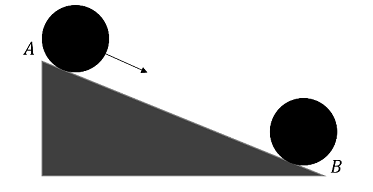a. 2
b. 1
c. 5
d. 7

When disc slides a1 = gsinθ

So,

$$\begin{array}{l}S = ut_{1}+\frac{1}{2}a_{1}t_{1}^{2}=\frac{1}{2}gsin\theta t_{1}^{2}\end{array}$$
…………(1)

When disc do pure rolling

$$\begin{array}{l}a_{2}=\frac{gsin\theta }{1+\frac{k^{2}}{R^{2}}}=\frac{gsin\theta }{1+1/2}=\frac{2}{3}gsin\theta\end{array}$$

So,

$$\begin{array}{l}S= ut_{2}+\frac{1}{2}a_{2}t_{2}^{2}=\frac{1}{2}\times \frac{2}{3}gsin\theta t_{2}^{2}\end{array}$$
——-(2)

From (1) and (2)

$$\begin{array}{l}\frac{t_{2}}{t_{1}}=\sqrt{\frac{3}{2}}\end{array}$$

So, x = 2

Question 7. An AC circuit consists of a series combination of an inductance L 1 mH, a resistance R = 111 and a capacitance C. It is observed that the current leads the voltage by 45°. Find the value of capacitance ‘C’ if the angular frequency of applied AC is 300 rad/s.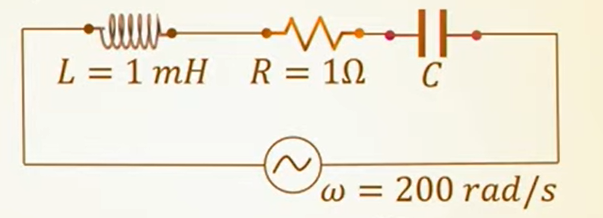a. 5.6 mF
b. 3.92 mF
c. 2.56 mF
d. 5.2 mF

$$\begin{array}{l}tan \Phi =\frac{X_{c}-X_{L}}{R}=\frac{\frac{1}{\omega C}-\omega L}{R}\end{array}$$
$$\begin{array}{l}1(1)=\frac{1}{300C}-300(1 x 10^{-3})\end{array}$$
$$\begin{array}{l}\frac{1}{300C}= 1+0.3 = 1.3\end{array}$$
$$\begin{array}{l}C = \frac{1}{300\times 1.3}=0.00256 F = 2.56 mF\end{array}$$

Question 8. An electron is projected into a magnetic field of B = 5 × 10−3 T and rotates in a circle of radius of R = 3mm. Find the work done by the force due to the magnetic field.

a. 0 J
b. 15 mJ
c. 14 mJ
d. 20 mJ

The work done by the force due to the magnetic field is 0.

Question 9. A charge Q is divided into q and (Q – q). If Q/q = x, such that the repulsion between them is maximum, find x.

a. 1
b. 2
c. 3
d. 4

As we know, F = (k(Q−q)q) /d2

For F to be maximum,

dE/dq = 0

⇒ Q − 2q = 0

⇒ Q/q = 2

⇒ x = 2

Question 10. Bird is flying in north-east direction with or v = 4√2 m/s with respect to the wind and the wind blowing from north to south with speed 1 m/s. Find the magnitude of the displacement of the bird in 3 sec.

a. 5 m
b. 15 m
c. 10 m
d. 20 m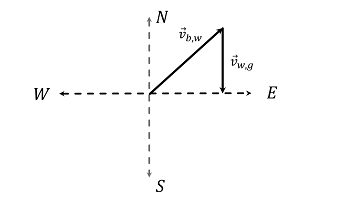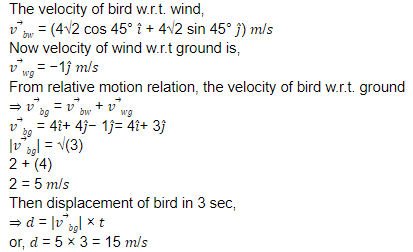Question 11. Deuteron and alpha particles having the same KE in a magnetic field. If the ratio of the radius of Deuteron and alpha particle is x√2. Then x =?

a. 5
b. 8
c. 3
d. 1

KE = B2q2r2/2m

As B and KE are the same

r2 ∝ m/q2 ⇒ r =√m/q

$$\begin{array}{l}\frac{r_{D}}{r_{al}}=\sqrt{\frac{m_{D}}{q_{al}}}\times \frac{q_{al}}{q_{D}}\end{array}$$
$$\begin{array}{l}\frac{r_{D}}{r_{al}}=\sqrt{\frac{2}{4}}\times \frac{2}{1}=\sqrt{2}\end{array}$$
$$\begin{array}{l}\Rightarrow \sqrt{2x}=\sqrt{2}\Rightarrow x =1\end{array}$$

Question 12. In the circuit shown, find the current through the Zener diode.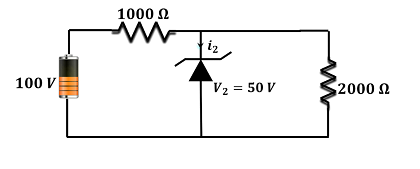a. 5 mA
b. 1 mA
c. 15 mA
d. 25 mA

Since 2000 Ω is parallel to the Zener diode

So, the current passing through it as shown in the circuit,

i3 =50/2000 = 25 mA

Potential difference across 1000 Ω,

𝑉1 = 100 − 50 = 50 𝑉

So, the electric current passing through it,

i1 = 50/1000 = 50 mA

So, current through Zener diode,

i2 = 50 − 25 = 25 mA

Question 13. If

$$\begin{array}{l}\vec{A}.\vec{B}=\vec{A}\times \vec{B}\end{array}$$
find
$$\begin{array}{l}\left | \vec{A}-\vec{B} \right |\end{array}$$

a. A – B
b.

$$\begin{array}{l}\sqrt{A^{2} + B^{2}-\sqrt{2AB}}\end{array}$$

c. A + B
d.
$$\begin{array}{l}\sqrt{A^{2} + B^{2}-\sqrt{2}AB}\end{array}$$

Since,

$$\begin{array}{l}\vec{A}.\vec{B}=\vec{A}\times \vec{B}\end{array}$$
$$\begin{array}{l}\Rightarrow \left | \vec{A} \right |\left | \vec{B} \right |cos\theta \Rightarrow \left | \vec{A} \right |\left | \vec{B} \right |sin\theta\end{array}$$

Angle between the vectors, θ = 450

Hence,

$$\begin{array}{l}\left | \vec{A}-\vec{B} \right |=\sqrt{A^{2}+B^{2}-2ABcos\theta } = \sqrt{A^{2} + B^{2}-\sqrt{2}AB}\end{array}$$

Question 14. An object moves from the earth’s surface to the surface of the moon. The acceleration due to gravity on the earth’s surface is 10 m/s2. Considering the acceleration due to gravity on the moon to be 1/6th times that of earth. If R be the earth’s radius and its weight be W and the distance between the earth and the moon is D. The correct variation of the weight W’ versus distance 𝑑 for a body when it moves from the earth to the moon is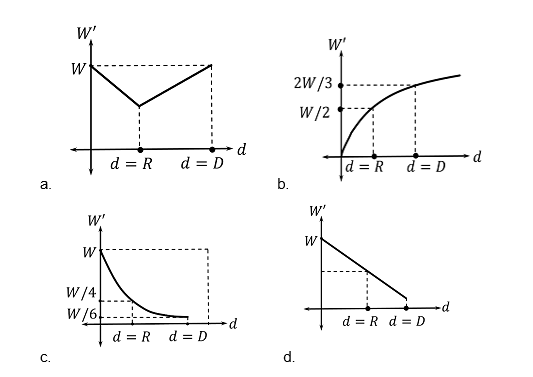At the earth’s surface, the weight of the body is, W = mg

At the moon, the distance of the body from the earth’s is d = D

At the moon’s surface, the value of acceleration due to gravity is g’ = g/6

⇒ W’ = W/6

From the relation of acceleration due to gravity at height d above earth’s surface,

$$\begin{array}{l}g’=\frac{gR^{2}}{(R+d)^{2}}—–(i)\end{array}$$

For d =0, ⇒g’ = g or W’ = W

At d = R,

⇒ g’ = gR2/4R2 = g/4

Or, W’ = mg/4 = W/4

At distance d = D, when the body reaches the surface of the moon

W’ = mg/6 = W/6

Since Eq.(i) suggests that variation of g with distance(d) is non-linear, hence the graph of

𝑊′ vs 𝑑 will be non-linear as well. Also, d ↑, W’ ↓

Thus, the correct variation is represented by the following graph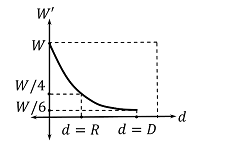Question 15. For an element decaying through simultaneous reaction, the half-life for the respective decaying path is 1400 𝑠 and 700 𝑠. Find the time taken when the number of atoms becomes N0/3 in the element sample. (N0 is the initial number of atoms in sample)

a. (1400/5) In 3
b. (1400/3) In 3
c. (1400/3)ln 2
d. (700/3)ln 2

N = N0et/τ

N0/3 = N0et/τ

Taking natural log on both sides,

$$\begin{array}{l}ln(\frac{1}{3})=(\frac{-t}{\tau })lne\end{array}$$
$$\begin{array}{l}-ln3=(\frac{-t}{\frac{1400}{3} })\times 1\end{array}$$

Therefore, t = (1400/3) ln3

Question 16: Consider a body of 800 kg moving with a maximum speed v on a road banked at θ= 300 given cos 300 = 0.87. Find the normal reaction on the body. Coefficient of friction μs = 0.2. [Take radius, r = 10 m]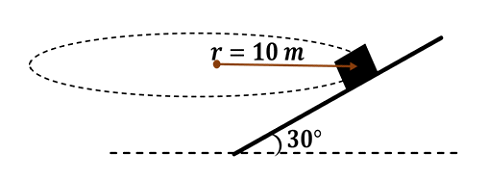a. 10. 4 kN
b. 12.6 kN
c. 11.6 kN
d. 8.3 kN

Since the circular motion is such that v = vmax the tendency of the body is to move up the

inclined plane

[fs]max = μsN = 0.2 N

Resolving along X and Y axis, we have N cos 300 = mg + [fs] max sin 300

⇒ N[0.87] = 8000 + 0.2𝑁 [1/2]

N[0.87 − 0.1] = 8000

N =8000/0.77 ≈10,400 newton

Question 17: A spring with natural length l0 has a tension T1 when its length is l1 and the tension is T2 when its length is l2. The natural length of spring will be:

a.

$$\begin{array}{l}\frac{T_{1}l_{2}-T_{2}l_{1}}{l_{1}-l_{2}}\end{array}$$

b.
$$\begin{array}{l}\frac{T_{2}l_{1}-T_{1}l_{2}}{T_{2}-T_{1}}\end{array}$$

c.
$$\begin{array}{l}\frac{T_{2}l_{2}-T_{1}l_{1}}{T_{1}-T_{2}}\end{array}$$

d.
$$\begin{array}{l}\frac{T_{2}l_{1}-T_{1}l_{2}}{T_{2}+T_{1}}\end{array}$$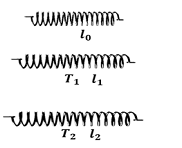Let the natural length be L0

Using hook’s law, Y= TL/AdL, where dL =L – L0

Case 1: When tension is T1 length of wire =L1

L1 – L0 = T1L0/AY ——-(1)

Case 2: Tension is T2 and length of wire = L2

L2 – L0 = T2L0/AY ——-(1)

Dividing both equations:

$$\begin{array}{l}\frac{T_{2}l_{1}-T_{1}l_{2}}{T_{2}-T_{1}}\end{array}$$

Question 18: A conducting rod of length l is moving perpendicular to the magnetic field. The rod moves from 0 to 2b while the field exists only from 0 to b. Find the graph for emf and power dissipated w.r.t x.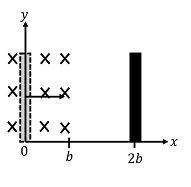From the given figure it is clear that the field exists from 0 to b only, therefore, the given conductor will experience a field only from 0 to b. Here the given conductor is moving in a

uniform magnetic field as long as field exists a constant emf (ε) will be induced in the conductor.

Induced emf in the conductor (ε) = Blv

Due to this emf current developed in the conductor as i = e.m.f/R = Blv/R

Power dissipation exists as long as emf exists in the conductor, p = i2R= (Blv)2/R

Hence, the graph of ε vs x and p vs x is as follows,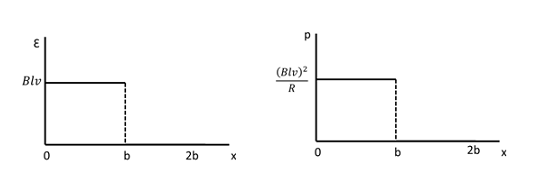Question 19: A travelling wave is found to have the displacement by y = 1/(1+x)2 at t = 0, after 3 sec the wave pulse is represented by equation y = 1/1+(1+x)2. The velocity of the wave is:

a. 1 m/s
b. ⅓ m/s
c. ⅔ m/s
d. ¼ m/s

Displacement of wave, Δx = 1 m

⇒ v x t = 1

t = 1/v

3 =1/v

v = ⅓ m/s

Question 20: A ball of charge to mass ratio of 8 μC/g is placed at a distance of 10 cm from the ball. An electric field of 100 N/m is switched on in the direction of the wall. Find the time period of its oscillations. Assume all collisions are elastic.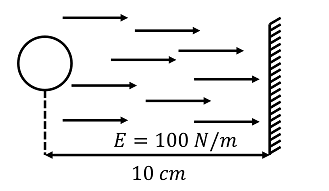a. 1 sec
b. 2 sec
c. 3 sec
d. 4 sec

$$\begin{array}{l}a =\frac{qE}{m}=\frac{8\times 10^{-6}}{10^{-3}}\times 100= 0.8m/s^{2}\end{array}$$

As the electric field is switched ON, the ball first strikes the wall and returns back.

One oscillation

Thus,

S = ut +(½)at12

0.1 = (½) × 0.8t12

t1 = (½) sec

Thus, time period,

T = 2 ×(½)

= 1 sec

Question 21: The wavenumber of the spectral line in the emission spectrum of hydrogen will be equal to 8/9 times of the Rydberg’s constant. Then the electron jumps from

a. 5 → 2
b. 5 → 3
c. 3 → 1
d. 4 → 2

$$\begin{array}{l}\bar{v}=Rz^{2}\left ( \frac{1}{n_{L}^{2}}-\frac{1}{n_{H}^{2}} \right )\end{array}$$

If nL = 1, nH = 3

$$\begin{array}{l}\bar{v}=R1^{2}\left ( \frac{1}{1^{2}}-\frac{1}{3^{2}} \right )=\frac{8}{9}R\end{array}$$

Question 22: A vehicle moving with velocity v and releasing the sound of frequency 400 Hz. Listening to the reflected sound from a wall of frequency 500 Hz. Find the velocity of vehicle v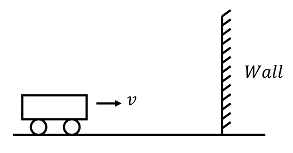a. 36.67 m/s
b. 30.12 m/s
c. 22.37 m/s
d. 20.25 m/s

$$\begin{array}{l}f’ =\left (\frac{v_{s}}{v_{s}-v} \right )f_{0}\end{array}$$

The reflected frequency received by man is

$$\begin{array}{l}f” =\left (\frac{v_{s}+v}{v_{s}} \right )\left ( \frac{v_{s}}{v_{s}-v} \right )f_{0}\end{array}$$
$$\begin{array}{l}f” =\left (\frac{v_{s}+v}{v_{s}-v} \right )f_{0}\end{array}$$

$$\begin{array}{l}500 =\left (\frac{330+v}{330-v} \right )400\end{array}$$

⇒ v = 330/9 = 36.67 m/s

Question 23: A particle of mass moving with a speed v collide elastically with the end of a uniform rod of mass M and length L perpendicularly as shown in the figure. If the particle comes to rest after collision, find the value of m/M.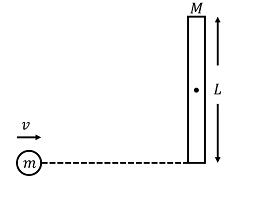a. 1/3
b. 1/2
c. 1/4
d. 1/5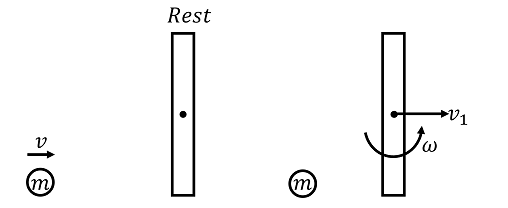Applying conservation of angular momentum about the Centre of mass of rod

$$\begin{array}{l}mv\left (\frac{L}{2} \right)=m\left ( \frac{L^{2}}{12} \right )\omega\end{array}$$
——–(i)

Applying linear momentum conservation

mv = Mv1……. (ii)

1 = [v1+ ω (L/ 2)] / v ……. (iii)

Putting v1 from (ii) and ωL from (i) in (iii)

$$\begin{array}{l}v =\frac{m}{M}v+\frac{6mv}{2M}\end{array}$$

⇒ 1 = 4m/M

⇒ m/M = 1/4

Question 24: Four planks are arranged in a lift going upwards with an acceleration of 0.2 m/s2 as shown in the figure. Find the normal reaction applied by the lift on 10 kg block: (g = 9.8 𝑚/𝑠2)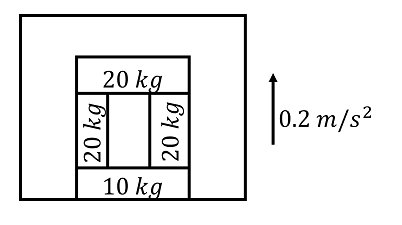a. 500
b. 700
c. 672
d. 800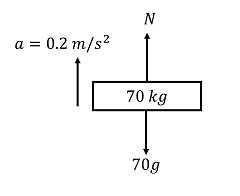N – 70g = 70 x 0.2

N = 70 (g + 0.2)

N = 700

Question 25: A body of mass m emits a photon of frequency 𝑣, then loss in its internal energy?

a.

$$\begin{array}{l}h\nu\end{array}$$

b.
$$\begin{array}{l}h\nu \left (1- \frac{h\nu }{2mc^{2}}\right )\end{array}$$

c.
$$\begin{array}{l}h\nu \left (1+ \frac{h\nu }{2mc^{2}}\right )\end{array}$$

d. Zero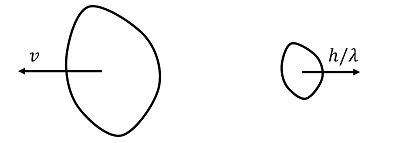$$\begin{array}{l}h\nu = \frac{c}{\lambda }\end{array}$$
$$\begin{array}{l}mv = \frac{h}{\lambda }=\frac{h\nu }{c}\end{array}$$

Loss of energy =

$$\begin{array}{l}\frac{1}{2}mv^{2}+h\nu\end{array}$$
$$\begin{array}{l}= \frac{1}{2m}\left ( \frac{h\nu }{c} \right )^{2}+h\nu\end{array}$$
$$\begin{array}{l}h\nu \left (1+ \frac{h\nu }{2mc^{2}}\right )\end{array}$$

Question 26: In a magnesium rod of area 3m2, current I = 5𝐴 is flowing angle of 600 from the axis of the rod. The resistivity of the material is 44 × 10−2 ohm x m. Find an electric field inside the rod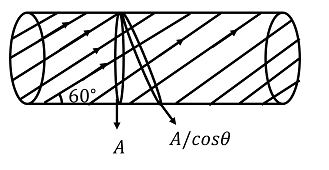a. 0.567
b. 0.367
c. 0.667
d. 0.767

$$\begin{array}{l}\frac{I}{A_{effective}}=\frac{E}{\rho }\end{array}$$
$$\begin{array}{l}E = \frac{\rho I}{A}cos 60^{0}=\frac{44\times 10^{-2}\times 5}{3\times 2}\end{array}$$